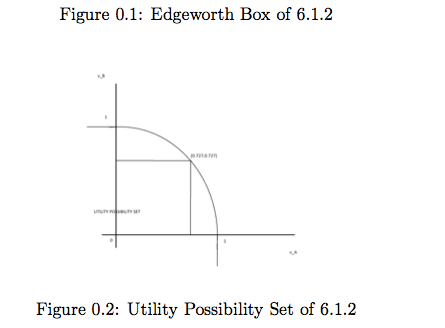# Modeling an Economy of Exchanges

Imagine a situation where people traded goods in a closed economy. In ancient times, this may have been an open-air market, where the community would gather together and trade items. The most important thing here is that there is no production, in that no new goods are being created. Although we can certainly model this, we will focus here on an exchange economy without production to begin.

To fix ideas, assume the economy consists of only everyone in this room, and the only goods in our utility functions are apples and bananas. Say someone comes in and arbitrarily gives us a bunch of different bundles, so maybe I have 0 bananas and 3 apples, maybe you have 5 bananas and 0 apples, etc. If our utility functions are dependent on both goods (for instance, u(x) = x1x2), immediately you can see we can be better off trading. If we didn’t, we would both have utility 0. The motivation behind general equilibrium thus follows. We need to find the allocations xθi in the end after trade, and the corresponding prices that dictate the trade. Generally, in computation, the prices are all that we want, and then we just do some algebra to get the allocations (because they were solved from first order maximization conditions).

We begin with n goods and Θ consumers. They are each endowed with goods, ωiθ, and we need to determine the equilibrium allocation and prices.

We begin with market clearing, and talk about the properties of an exchange economy. We will state propositions without their proofs for brevity.Some interpretation: It basically shows that the price of one market does not matter. If n − 1 markets are balanced, the nth one is as well. Competitive markets have relative prices. This is why we can denote one as the numeraire, i.e. setting pi = 1 for some i.

Pareto efficiency means no one can be made better off without someone being worse off. Immediately you can see that we avoid any talk about equity considerations, which is the main drawback of this definition. If I am the government, and I were to give everyone here some amount of money, giving someone everything and giving everyone a little bit are both pareto efficient.

Definition 0.2. An allocation is pareto efficient if xˆ bundle such that xˆ ≽ x for all θ and xˆ ≻ x for at least one θ.

How does this relate to general equilibrium?

Theorem 0.3 (First Welfare Theorem). Competitive Equilibria are Pareto efficient.

So competitive equilibria are efficient, and Pareto efficiency also implies equated MRSs, which is characteristic of a competitive equilibrium. Note efficiency is not enough to say something is a competitive equilibrium, but it gives a good start.

Theorem 0.4 (Second Welfare Theorem). Consider a feasible efficient allocation x. We can rearrange endowments to ensure x is a competitive equilibrium allocation.

Let’s work anexample to see this exchange economy in action.

Example 0.5 (No Firms). Consider the Edgeworth box economy where the endowment of consumer A is(1,0) and the endowment of consumer B is (0,1). For each of the following three cases, find and sketch the set of Pareto optimal allocations and the utility possibility set and find the allocations that maximize the sum of the utilities of the two consumers.Solution. We employ a systematic approach. We must solve the equation MRSA =MRSB

Since the endowment of each commodity is one, this equation becomes

In our example, this becomesThe point (0.727,0.727) on the frontier may be found by letting xA1 = 1/3. Then xA2 = 2/3 so thatxB1 = 2/3 and xB2 = 1/3. At these values for the allocation, vA = vB = 0.727. The concavity of the utility possibility frontier is a consequence of the concavity of the utility functions. The symmetry of the utility possibility set with respect to the two axes follows from the symmetry of the problem. Therefore if some allocation is possible, switching the two is also feasible and the utility levels achieves are symmetric. Therefore, the sum-utility-maximizing allocation is where the allocation is ((1/3, 2/3), (2/3, 1/3)).

Justin Y. is our incredible math and economics tutor in NYC, Brooklyn and Queens. He is ready to answer any questions and start working with you. Please call 646-407-9078.